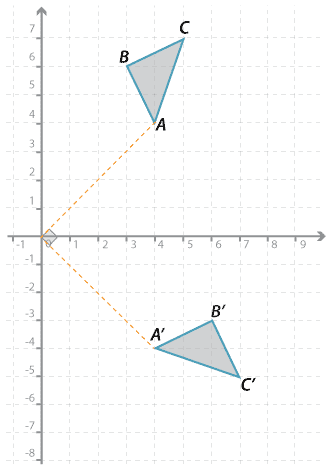#### Rotating by 90 ° in a clockwise direction about the originDetailed description

We see:

$$A(4, 4) \rightarrow A^\prime (4, −4); B(3, 6) \rightarrow B^\prime (6, −3); C(5, 7) \rightarrow C^\prime (7, −5)$$.

#### Question 4

The rectangle $$ABCD$$ is rotated clockwise by 90 ° around the origin to form rectangle $$A^\prime B^\prime C^\prime D^\prime$$.

The coordinates of each of the vertices of $$ABCD$$ are as follows:

$$A(1, 6), B(1, 9), C(2, 9), D(2, 6)$$.

Give the $$x$$-coordinate and the $$y$$-coordinate of:

$$x$$ = $$y$$ = Submit answer
a $$A^\prime$$ ( , ).
b $$B^\prime$$ ( , ).
c $$C^\prime$$ ( , ).
d $$D^\prime$$ ( , ).

Place answers in both checkboxes before submitting.